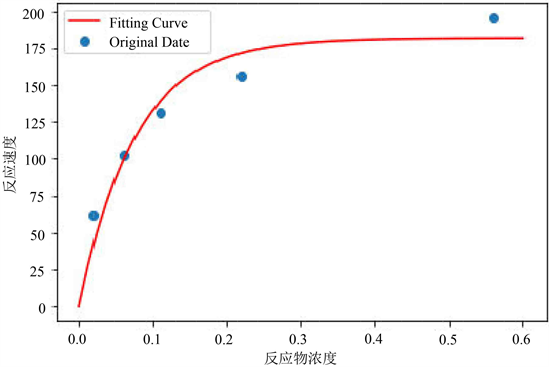# 一类新的无参数填充函数及其在最小二乘法中的应用A New Type of Non-Parameter Filled Function and Its Application in Least Squares

• 全文下载: PDF(840KB)    PP.753-761   DOI: 10.12677/AAM.2019.84085
• 下载量: 221  浏览量: 317   科研立项经费支持

In this paper, a novel non-parameter filled function for solving general constrained global optimi-zation problem is proposed. Then the theoretical properties of the function are argued and corre-sponding algorithm is given in this paper. Numerical experiments using the Python programming language and comparison with previous results show that the proposed filled function algorithm is not only effective but also works better when dealing with certain function. Furthermore, the algorithm is tentatively combined with the treatment of the reaction rate and reactant concentra-tion of the enzymatic reaction of chemical experiments, indicating that the algorithm also has good adaptability in practical cases.

1. 引言

2. 新的无参数填充函数

$\left(P\right)\left\{\begin{array}{l}\mathrm{min}f\left(x\right),\\ \text{s}\text{.t}\text{.}\text{\hspace{0.17em}}x\in {R}^{n}.\end{array}$ (2.1)

$\left\{\begin{array}{l}\mathrm{min}f\left(x\right),\\ \text{s}\text{.t}\text{.}\text{\hspace{0.17em}}x\in X\end{array}$ (2.2)

1) ${x}^{*}$$p\left(x,{x}^{*}\right)$ 的一个严格局部极大点， $f\left(x\right)$ 在点 ${x}^{*}$ 处的盆谷 ${B}^{*}$ 成为 $p\left(x,{x}^{*}\right)$ 的峰的一部分。

2) $p\left(x,{x}^{*}\right)$ 在比 ${B}^{*}$ 高的盆谷里没有平稳点，即 $\nabla p\left(x,{x}^{*}\right)\ne 0$

3) 如果存在比 ${B}^{*}$ 低的盆谷 ${B}_{1}^{*}$ ，则在 ${x}^{\prime }$${x}^{*}$ 的连线上极小化 $p\left(x,{x}^{*}\right)$ 得到极小点 ${x}^{″}\in {B}^{*}$ ，其中， $N\left({x}_{1}^{*},{\delta }_{1}\right)\left({\delta }_{1}>0\right)，{x}^{\prime }\in N\left({x}_{1}^{*},{\delta }_{1}\right)$

1) ${x}^{*}\in {B}^{*}$

2) 对于任意一点 $x\in {B}^{*}$ 使得 $x\ne {x}^{*}$$f\left(x\right)>f\left({x}^{*}\right)$ ，存在一条从 $x$ 到c的下降路径。

$\begin{array}{r}\hfill p\left(x,{x}^{*}\right)=-\mathrm{ln}\left(1+{‖x-{x}^{*}‖}^{2}\right)\left(f\left(x\right)-f\left({x}^{*}\right)\right).\end{array}$ (2.3)

$\begin{array}{c}p\left(x,{x}^{*}\right)=-\mathrm{ln}\left(1+‖x-{x}^{*}‖\right)\left(f\left(x\right)-f\left({x}^{*}\right)\right)\\ <0=p\left({x}^{*},{x}^{*}\right)\end{array}$

$f\left(x\right)=f\left({x}^{*}\right)+{\left(x-{x}^{*}\right)}^{T}\nabla f\left(x\right)+o\left(‖x-{x}^{*}‖\right)$

$f\left(x\right)-f\left({x}^{*}\right)={\left(x-{x}^{*}\right)}^{T}\nabla f\left(x\right)+o\left(‖x-{x}^{*}‖\right)>0$

$\nabla p\left(x,{x}^{*}\right)=-\left[\frac{2\left(x-{x}^{*}\right)}{1+{‖x-{x}^{*}‖}^{2}}\left(f\left(x\right)-f\left({x}^{*}\right)\right)+\mathrm{ln}\left(1+{‖x-{x}^{*}‖}^{2}\right)\nabla f\left(x\right)\right]$

$\begin{array}{c}\nabla p{\left(x,{x}^{*}\right)}^{T}\left(x-{x}^{*}\right)=\left[\frac{2{‖x-{x}^{*}‖}^{2}}{1+{‖x-{x}^{*}‖}^{2}}\left(f\left(x\right)-f\left({x}^{*}\right)\right)+\mathrm{ln}\left(1+{‖x-{x}^{*}‖}^{2}\right)\nabla f{\left(x\right)}^{T}\left(x-{x}^{*}\right)\right]\\ <0\end{array}$

$p\left(x,{x}^{*}\right)=-\mathrm{ln}\left(1+{‖x-{x}^{*}‖}^{2}\right)\left(f\left(x\right)-f\left({x}^{*}\right)\right)<0$

$p\left({x}^{\prime },{x}^{*}\right)=-\mathrm{ln}\left(1+{‖{x}^{\prime }-{x}^{*}‖}^{2}\right)\left(f\left({x}^{\prime }\right)-f\left({x}^{*}\right)\right)>0$

3. 填充函数算法

${x}^{*}+\delta \left(1,0\right),{x}^{*}+\delta \left(0,1\right),{x}^{*}-\delta \left(1,0\right),{x}^{*}-\delta \left(0,1\right)$

4. 数值实验

4.1. 测试函数

1) 6-Hump Back Camel函数 

$\left\{\begin{array}{l}\mathrm{min}f\left(x\right)=4{x}_{1}^{2}-2.1{x}_{1}^{4}+\frac{1}{3}{x}_{1}^{6}-{x}_{1}{x}_{2}-4{x}_{2}^{2}+4{x}_{2}^{4}\\ \text{s}\text{.t}\text{.}\text{\hspace{0.17em}}-3\le {x}_{1},{x}_{2}\le 3\end{array}$

2) Restrign函数 

$\left\{\begin{array}{l}\mathrm{min}f\left(x\right)={x}_{1}^{2}+{x}_{2}^{2}-\mathrm{cos}\left(18{x}_{1}\right)-\mathrm{cos}\left(18{x}_{2}\right)\\ \text{s}\text{.t}\text{.}\text{\hspace{0.17em}}-1\le {x}_{1},{x}_{2}\le 1\end{array}$

3) 文献  中的一个二维函数(c = 0.05)

$\left\{\begin{array}{l}\mathrm{min}f\left(x\right)={\left[1-2{x}_{2}+c*\mathrm{sin}\left(4\text{π}{x}_{2}\right)-{x}_{1}\right]}^{2}+{\left[{x}_{2}-0.5\mathrm{sin}\left(2\text{π}{x}_{1}\right)\right]}^{2}\\ \text{s}\text{.t}\text{.}\text{\hspace{0.17em}}-10\le {x}_{1},{x}_{2}\le 10\end{array}$

4.2. 数值结果

$k$ ：求解第 $k$ 次局部极小点的迭代步数；

${x}_{k}$ ：满足 ${x}_{k}\in X$ 的第 $k$ 次初始点；

${x}_{k}^{*}$ ：第 $k$ 个局部极小点；

$f\left({x}_{k}^{*}\right)$ ：第 $k$ 个局部极小点的目标函数值；

$\text{iter}$ ：算法迭代步数；

${x}^{*}$ ：算法最终得到的全局极小点；

$f\left({x}^{*}\right)$ ：算法最终得到的全局极小值。Table 1. Original value x = ( 1 , 2 ) TTable 2. Original value x = ( 1 , 1 ) TTable 3. c = 0.05 ，Original value x = ( 6 , − 2 ) TTable 4. Compared with paper 

5. 填充函数在实验数据处理中的应用

5.1. 背景和问题Table 5. Reaction rate and substrate concentration data in puromycin experimentsTable 6. Average the data after treatment with scorpion toxin

5.2. 分析与假设

$y=f\left(x,\beta \right)={\beta }_{1}\left(1-{e}^{-{\beta }_{2}x}\right)$

$\begin{array}{c}\mathrm{min}f\left(x\right)={\underset{i=1}{\overset{n}{\sum }}\left({y}_{i}-{\stackrel{^}{y}}_{i}\right)}^{2}\\ =\underset{i=1}{\overset{n}{\sum }}{\left({\beta }_{1}\left(1-{e}^{-{\beta }_{2}{x}_{\text{​}}}\right)-{\stackrel{^}{y}}_{i}\right)}^{2}\end{array}$Figure 1. Fitting curve

6. 结语

  Ge, R.P. (1990) A Filled Function Method for Finding a Global Minimizer of a Function of Several Variables. Mathe-matical Programming, 46, 191-204. https://doi.org/10.1007/BF01585737  Ge, R.P. and Qin, Y.F. (1987) A Class of Filled Functions for Finding Global Minimizers of a Function of Several Variables. Journal of Optimization Theory and Applications, 54, 241-252. https://doi.org/10.1007/BF00939433  Zhang, L.S., Ng, C.-K., Li, D. and Tian, W.W. (2004) A New Filled Function Method for Global Optimization. Journal of Global Optimization, 28, 17-43. https://doi.org/10.1023/B:JOGO.0000006653.60256.f6  Lin, Y.J. and Yang, Y.J. (2010) Filled Func-tion Method for Nonlinear Equations. Journal of Computational and Applied Mathematics, 234, 695-702. https://doi.org/10.1016/j.cam.2010.01.007  王伟祥, 尚有林, 张连生. 约束全局优化问题的一个单参数填充函数方法[J]. 工程数学学报, 2008, 25(5): 795-803.  Zhang, Y. and Xu, Y.T. (2009) A One-Parameter Filled Function Method Applied to Nonsmooth Constrained Global Optimization. Computers & Mathematics with Applications, 58, 1230-1238. https://doi.org/10.1016/j.camwa.2009.07.038  Yang, Y.J. and Shang, Y.L. (2006) A New Filled Function for Unconstrained Global Optimizaton. Applied Mathematics and Computation, 173, 501-512. https://doi.org/10.1016/j.amc.2005.04.046  He, S.X., Chen, W.L. and Wang, H. (2011) A New Filled Function Algorithm for Constrained Global Optimization Problems. Applied Mathematics and Computation, 217, 5853-5859. https://doi.org/10.1016/j.amc.2010.12.070  冉慧, 宋雪. 求解约束优化问题的无参数填充函数算法[J]. 重庆理工大学学报(自然科学版), 2013, 27(5): 132-136.  Lin, H.W., Wang, Y.P., Wang, X.L. and Gao, Y.L. (2012) A New Filled Function Method for Global Optimization with Box Constraint. Journal of Information & Computational Science, 10, 2843-2853.  An, L., Zhang, L.S. and Chen, M.L. (2004) A Parameter-Free Filled Function for Global Unconstrained Optimization. Journal of Shanghai University (English Edition), 215, 3610-3619.  姜启源, 谢金星, 叶俊. 数学模型 [M]. 第四版. 北京: 高等教育出版社, 2011.